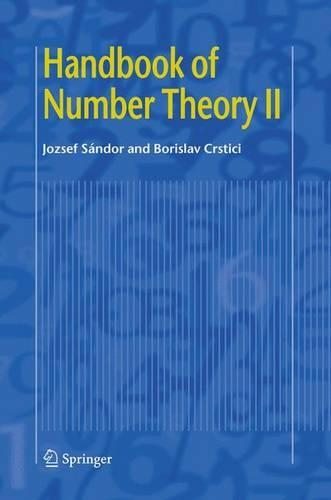•# Handbook of Number Theory II (Hardback)

(author), (author)
£179.99
Hardback 637 Pages / Published: 10/01/2005
• We can order this

Usually dispatched within 15 working days

This handbook focuses on some important topics from Number Theory and Discrete Mathematics. These include the sum of divisors function with the many old and new issues on Perfect numbers; Euler's totient and its many facets; the Moebius function along with its generalizations, extensions, and applications; the arithmetic functions related to the divisors or the digits of a number; the Stirling, Bell, Bernoulli, Euler and Eulerian numbers, with connections to various fields of pure or applied mathematics. Each chapter is a survey and can be viewed as an encyclopedia of the considered field, underlining the interconnections of Number Theory with Combinatorics, Numerical mathematics, Algebra, or Probability Theory.

This reference work will be useful to specialists in number theory and discrete mathematics as well as mathematicians or scientists who need access to some of these results in other fields of research.

Publisher: Springer-Verlag New York Inc.
ISBN: 9781402025464
Number of pages: 637
Weight: 2370 g
Dimensions: 235 x 155 x 34 mm
Edition: 2004 ed.

MEDIA REVIEWS

From the reviews:

"It is the aim of this monograph to give, in an encyclopedic manner, surveys on some topics of Number Theory with many hints to the literature. ... The reviewer thinks that this monograph is a great help to number theorists ... ." (Wolfgang Schwarz, Zentralblatt MATH, Vol. 1079 (5), 2006)

"This book is a compendium of results in certain areas of number theory ... . This volume can be quite useful for researchers ... and each chapter ends with a large list of references." (Don Redmond, Mathematical Reviews, Issue 2005 k)